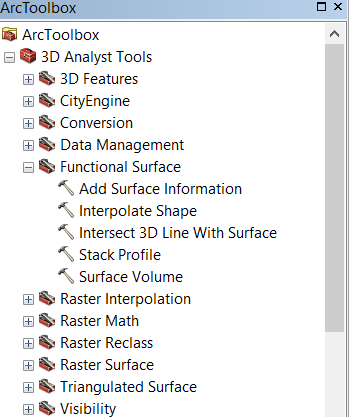# measure distance between points, - taking terrain elevatin into account.

2989
3
02-11-2016 05:37 AM
New Contributor

Ok I'm changing this, it just got simpler.  Yes- and I have 10.1 advanced, and enterprise server- with spatial analyst, 3d and network analyst.

They pass us a location, that will be a point from one of a series of preexisting layers (which may include lat long or mailing address, but not generally. Generally it will be a preexisting point for a point layer.

We need to measure a straight line from that - selected point - to a bunch of points in 1 other layer.

That's it. - looking for an output of a table of distances and point information.

Tags (3)
3 Replies
Regular Contributor III

This sounds like a Spatial Analyst​ function. Do you have a spatial analyst license?

edit:

byMVP Esteemed Contributor

EDIT

It appears the question was edited to simplify the problem to ignore terrain or 3D distance so ...

--------------------- to original question

You need the 3D analyst

Depending how you want to do it,you can produce graphs using the 3D toolbar or you can use one of the tools in arctoolbox perhaps the Add Surface Information tool, if you already have shapes, particularly polylines.byMVP Honored Contributor

If you're only interested in the straight-line 3d distance between points, you can use good-old math to figure it out without 3D Analyst:

`>>> sr = arcpy.Describe("valley_pt_elev").spatialReference # spatial ref... with arcpy.da.SearchCursor("valley_pt_elev","SHAPE@",spatial_reference=sr) as sCursor: # get original point geometry...     for sRow in sCursor:...         orig_point = sRow.centroid... with arcpy.da.UpdateCursor("mtn_pnts_elev",["SHAPE@","dist_3d"],spatial_reference=sr) as uCursor: # loop through other points...     for uRow in uCursor:...         point = uRow.centroid...         dx = point.X - orig_point.X...         dy = point.Y - orig_point.Y...         dz = point.Z - orig_point.Z...         hor_dist = math.sqrt(math.pow(dx,2) + math.pow(dy,2)) # calculate horizontal distance...         dist_3d = math.sqrt(math.pow(dz,2) + math.pow(hor_dist,2)) # calculate 3d distance...         print(dx,dy,dz,dist_3d,hor_dist,uRow.distanceTo(orig_point)  )      ...         uRow = dist_3d ...         uCursor.updateRow(uRow) # update 3d distance field`